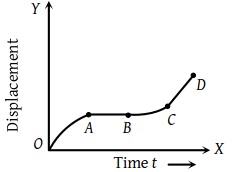The graph between the displacement x and time t for a particle moving in a straight line is shown in figure. During the interval $OA,\text{\hspace{0.17em}}AB,\text{\hspace{0.17em}}BC$ and CD, the acceleration of the particle isOA AB BC CD (1) + 0 + + (2) – 0 + 0 (3) + 0 – + (4) – 0 – 0

Concept Videos :-

#20-Graph x vs t
#21- v vs t and a vs t graph
#22-Solved-Examples-12
#23-Solved-Examples-13
#24-Solved-Examples-14
#25-Solved-Examples-15

Concept Questions :-

Graphs

(2) Region OA shows that graph bending toward time axis i.e. acceleration is negative.

Region AB shows that graph is parallel to time axis i.e. velocity is zero. Hence acceleration is zero.

Region BC shows that graph is bending towards displacement axis i.e. acceleration is positive.

Region CD shows that graph having constant slope i.e. velocity is constant. Hence acceleration is zero.

Difficulty Level:

• 34%
• 50%
• 14%
• 4%